# Drawing Fractals with L-Systems in D3.js

Categories:

This is the development post for my L-System project, where I ‘ll show you how to draw all kinds of fractals in D3.js. If you haven’t seen it, be sure to check it out first, or at least let the following fractals we are going to build get you excited.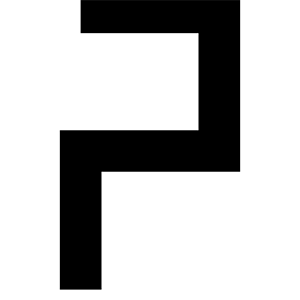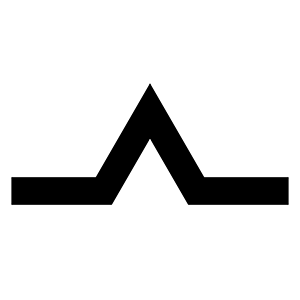## Preliminaries

### What is an L-System?

A Lindenmayer-System (L-System) is a set of string rewriting rules. If you know what a Context Free Grammar (CFG) is, then an L-System is basically the same, however at each iteration you have to apply every applicable production (rewriting rule). If you don’t know anything about formal grammars, you basically start with a string of letters (taken from your “Alphabet”), and have rules how to transform a letter into different letters. Let’s look at an example:

``````A // this is the axiom (start symbol of your grammar)
A=+B-A-B+ // production for A
B=-A+B+A- // production for B``````

The start of your derivation is the symbol A, and it has two productions, one for A and one for B. As you can see, there can be letters that don’t have a production, like ’+’ and ’-’, these simply stay unchanged in the derivations. Let’s look at the first three iterations:

``````Iteration 0: A // start symbol
Iteration 1: +B-A-B+
Iteration 2: + -A+B+A- - +B-A-B+ - -A+B+A- +
Iteration 3: +-+B-A-B++-A+B+A-++B-A-B+--+-A+B+A--+B-A-B+--A+B+A-+--+B-A-B++-A+B+A-++B-A-B+-+``````

For the first iteration we simply apply the first rule for ‘A’, in the second iteration we have to apply the rules for B,A,B again. The derivations can grow big quite fast, usually exponential as a single letter gets replaced by two or more letters in each iteration.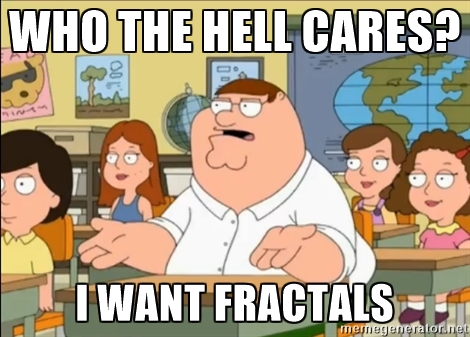### From L-Systems to Fractals

Maybe surprisingly, L-Systems are one of the best ways to draw fractals, due to their recursive nature. Lindenmayer invented them to study the growth and self-similarity of plants. To get from an L-System to a fractal, you imagine you start with a pen (or a turtle) on a piece of paper, and interpret the string’s letters in a predefined way. In our example above, if A and B both mean draw a straight line of constant length forward, and +/- mean turn left/right by 60°, then the resulting drawings from the strings of our iterations approximate the Sierpinski triangle.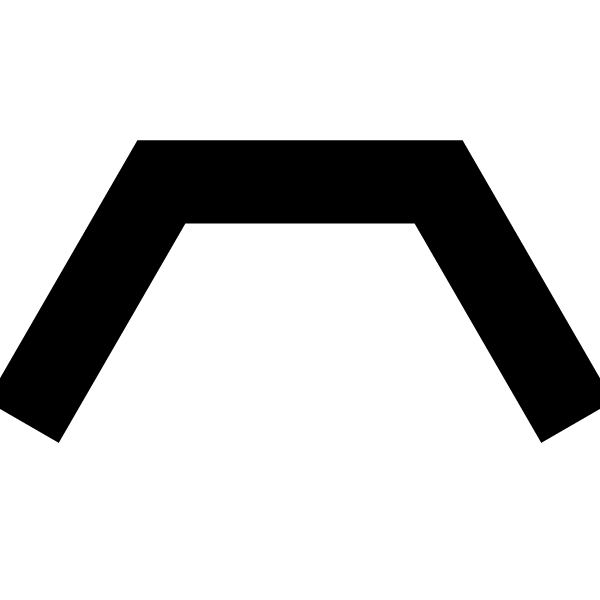To study fractal plants, we can look at this L-System:

``````X // start symbol
X=F-[[X]+X]+F[+FX]-X
F=FF``````

We will interpret F again as draw a straight line of constant size forward, and +/- by turn left/right by 25°. For complex fractals, we additionally need [ which stores the current position and angle on a stack, and ] pops it. The result is this beautiful fractal plant.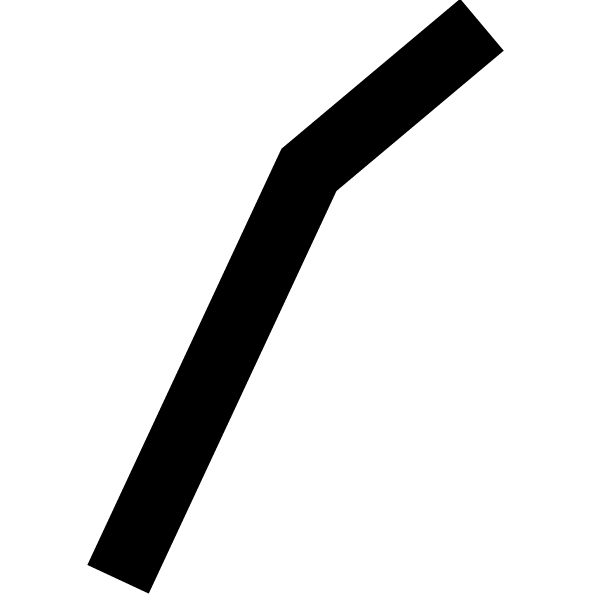## Implementation

The implementation of the L-System parser and the Turtle Graphics is quite simple and only needs a few lines of code. For the UI and storing state of the application, I used React and Redux, while D3.js renders the fractals in a `svg`. The full source code is available on GitHub, the most interesting parts are probably the L-System Parser and the Turtle Drawing SVG System

Play around with my implementation here, change the productions to create some interesting fractals, and share them with me in the comments.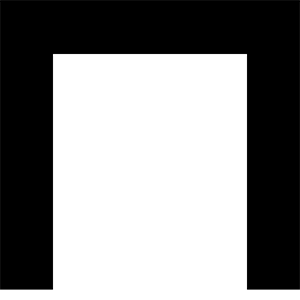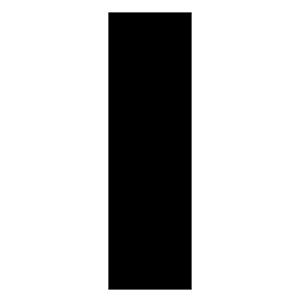# Hi, I'm Christoph Michel 👋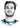I'm a , , and .

I mostly do and help small to mid-sized teams from all over the world 🌎 on both short and long-term projects on an independent contractor basis.

I strive for efficiency ⏱️ and therefore track many aspects of my life.